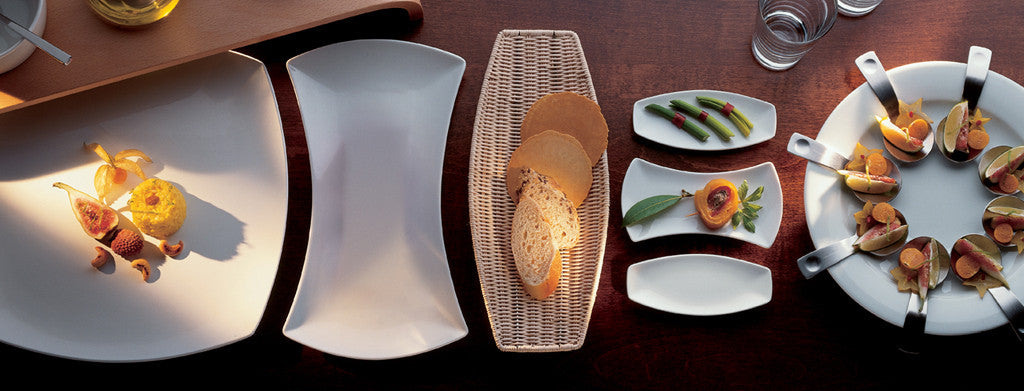Shopping Cart

### Refine

View all

#### Price# Crockery£8.83 each (ex VAT)£17.40 each (ex VAT)£13.06 each (ex VAT)£10.48 each (ex VAT)£15.08 each (ex VAT)£12.10 each (ex VAT)£10.30 each (ex VAT)£13.06 each (ex VAT)£10.48 each (ex VAT)£8.90 each (ex VAT)£12.10 each (ex VAT)£10.30 each (ex VAT)£112.92£77.28£47.97 each (ex VAT)£31.95 each (ex VAT)£14.47 each (ex VAT)£10.12 each (ex VAT)£10.12 each (ex VAT)£10.12 each (ex VAT)£10.12 each (ex VAT)£10.12 each (ex VAT)£10.12 each (ex VAT)£10.12 each (ex VAT)£9.89 each (ex VAT)£19.77 each (ex VAT)£14.80 each (ex VAT)£21.72 each (ex VAT)£16.48 each (ex VAT)£9.44 each (ex VAT)£21.62 each (ex VAT)£16.30 each (ex VAT)£15.34 each (ex VAT)£12.44 each (ex VAT)£8.86 each (ex VAT)£7.87 each (ex VAT)£6.80 each (ex VAT)£6.39 each (ex VAT)£16.68 each (ex VAT)£15.72 each (ex VAT)£14.09 each (ex VAT)£33.67 each (ex VAT)£29.70 each (ex VAT)£25.93 each (ex VAT)£21.03 each (ex VAT)£23.70 each (ex VAT)£24.87 each (ex VAT)£16.39 each (ex VAT)